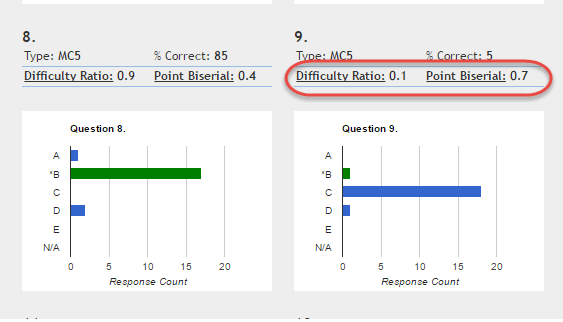# What do Difficulty Ratio and Point Biserial mean?

Follow

Not what you were looking for? Search here.

The Item Analysis Report shows statistics for each question on the assessment. Most are self-explanatory, like the type of question, the percent of students who got the correct answer, and the bar graph showing how many students chose each possible answer.

This article will explain the other two values: Difficulty Ratio and Point Biserial.Difficulty Ratio

In simple terms, this is a rank of how easy the question is. The range goes from 0.1 to 1, in tenths-of-a-point increments (0.1, 0.2, etc.).

0.1 = Very difficult. Very few students answered it correctly.

1 = Very easy. All students answered it correctly.

Note in the above images that question 8 got a Difficulty Ratio of .9 because a high number of students got it right, while question 9 got a Difficulty Ratio of 0.1 because very few got it right.

Point Biserial

This value shows the correlation of any given student's performance on a question with their overall performance on the assessment. In other words, is this question a good predictor of overall performance on the assessment?

This indicator can be especially useful for determining if a question is good at assessing the general understanding. For an assessment that only covers one standard, this could help you determine that the way a question is written is effective at assessing that standard.

The range of Point Biserial goes from -1 to 1.

1 = Highest correlation between how students answered this question and how they performed overall on the assessment. This could indicate that the question is a "good question" for assessing general understanding.

0 = No correlation between how students answered this question and how they performed on the assessment. Note: It may be that all students got it either correct or incorrect, and a correlation could not be accurately determined.

-1 = Inverse correlation between how students answered this question and how they performed on the assessment. This could indicate that there is a problem with the test key, the wrong correct answer is designated for this question, or the question is written in a misleading way.

Note that in the image above, question 8 got a Point Biserial of 0.4, indicating "somewhat" of a correlation between how students performed on this question and how they performed on the assessment, while question 9 got a 0.7, indicating a higher correlation.